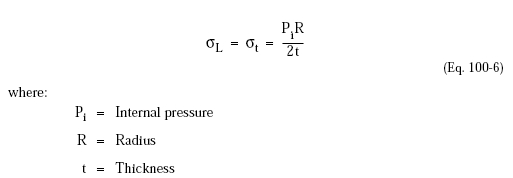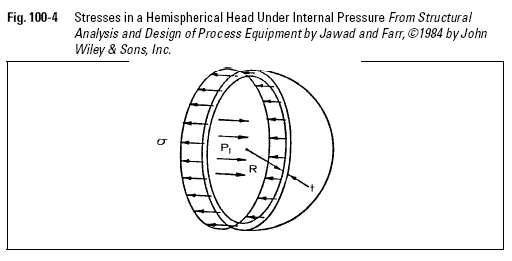﻿ Pressure Vessel Spherical Shells and Hemispherical Heads Under Internal Pressure | Oil & Gas Industry Technology Updates

## Pressure Vessel Spherical Shells and Hemispherical Heads Under Internal Pressure

A sphere is the ideal shape for containing internal pressure because: (1) the loads developed in the shell are lower than for any other shape; (2) a sphere has the lowest surface area for the volume contained; and (3) a sphere will have the lowest weight. However, because spheres are more difficult to fabricate, hemispherical heads fitted to cylindrical shells are more often used for large diameter or high pressure vessels.

The stress determined from the diagram in Figure 100-4 is,Note that the longitudinal stress (QL) and hoop stress (Qt) are the same because of the uniform geometry of the sphere. Therefore, the maximum stress in a sphere is half that for a cylinder of the same diameter. Equation 100-6 assumes a uniform stress distribution through the thickness, and is adequate for relatively thin heads. As the wall thickness increases with respect to the radius, however, the assumption that the stress is uniformly distributed through the wall is invalid. A “thick sphere” equation must then be used, having the maximum stress at the inside surface, similar to the equations for a thick-walled cylinder. Like the stress equations for cylindrical shells, the ASME Code uses an approximation of the thick-sphere equation and applies it to all thicknesses.Categories: Process Design | Leave a comment